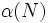# Normal-homomorph-containing implies strictly characteristic

This article gives the statement and possibly, proof, of an implication relation between two subgroup properties. That is, it states that every subgroup satisfying the first subgroup property (i.e., normal-homomorph-containing subgroup) must also satisfy the second subgroup property (i.e., strictly characteristic subgroup)
View all subgroup property implications | View all subgroup property non-implications
Get more facts about normal-homomorph-containing subgroup|Get more facts about strictly characteristic subgroup

## Statement

### Statement with symbols

Suppose$N$ is a normal-homomorph-containing subgroup of a group$G$: for any homomorphism$\varphi:N \to G$ such that$\varphi(N)$ is normal in$G$, we have$\varphi(N) \le N$.

Then,$N$ is a strictly characteristic subgroup of$G$: for any surjective endomorphism$\alpha$ of$G$,$\alpha(N) \le N$.

## Facts used

1. Normality satisfies image condition: The image of a normal subgroup under a surjective homomorphism is a normal subgroup of the image.

## Proof

Given: A normal subgroup$N$ of a group$G$ such that whenever$\varphi:N \to G$ is a homomorphism such that$\varphi(N)$ is normal in$G$, we have$\varphi(N) \le N$. A surjective endomorphism$\alpha$ of$G$.

To prove:$\alpha(N) \le N$.

Proof:

1. (Given data used:$N$ is normal in$G$,$\alpha$ is a surjective endomorphism):$\alpha(N)$ is a normal subgroup of$G$: By fact (1), the image$\alpha(N)$ is a normal subgroup of$\alpha(G)$. By surjectivity, we have$\alpha(G) = G$, so$\alpha(N)$ is normal in$G$.
2. (Given data used:$N$ is normal-homomorph-containing):$\alpha(N) \le N$: Let$\varphi:N \to G$ be the restriction of$\alpha$ to$N$. Then$\varphi(N) = \alpha(N)$ by definition, and by step (1),$\varphi(N)$ is normal in$G$. Since$N$ is normal-homomorph-containing, we get$\varphi(N) \le N$, so$\alpha(N) \le N$.